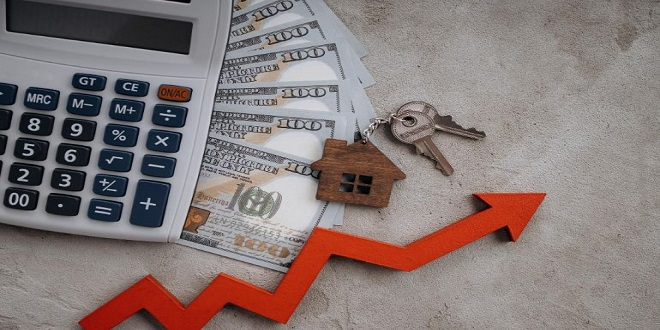# What is an FD Interest Calculator?

Thomas Edison once said, “Opportunity is missed by most people because it is dressed in overalls and looks like work.”

Fixed deposits are a secure way to invest your money these days. They are also known as term deposits or FDs. The investor is expected to deposit a lump sum amount for a predetermined tenure at a fixed interest rate in this method of investment. Following the maturity of the FD, the investor receives the original investment amount plus compound interest accumulated over the years.Source: Shutterstock

FD might sound like a complicated subject but in reality, it is not that tricky. Opportunities aren’t easy to come by, therefore knowing about the best method of investment ensures you don’t miss out on the possible ones. It will assist you to make the right financial decision and understanding how an FD interest calculator works can be the first step in doing so.

What is an FD Interest Calculator?

An FD Interest Calculator is a helpful tool for calculating interest returns on your investment. It considers the principal investment, the interest rate, and the duration of the fixed deposit. An FD Interest calculator is a simple tool that allows you to compare which banks offer the best interest rate on FD.

Wealth management can be a difficult concept to grasp, especially when it comes to investing a large sum of money all at once. Using an FD Interest calculator, you can calculate the compound interest you would earn on your investment over terms ranging from 7 days to 10 years. It will ensure that you are making the best investment decision possible.

How to Determine FD Interest Amount

An FD Interest Calculator calculates the investment’s maturity value based on three factors. These variables differ from person to person because they must be decided and entered by the people themselves.

Formula to calculate FD maturity amount –

A = P * (1+ r/n) n*t,

A = Value of Maturity

I = A – P

P = principal sum

r = interest rate

n = compound interest rate

t = number of years

I = total interest earned

Here is an example to better understand how an FD maturity amount is calculated –

Suppose you deposit Rs. 50,000 that is held for three years at a quarterly compounding interest rate of 10% then the interest earned at maturity would be:

A= 50,000 {1 + (0.1/4)} ^ (4 * 3)

A = 50,000 (1 + 0.025) ^ (12)

A = 50,000 (1.025) ^ (12)

= Rs. 67,244 (approximately)

CI = Maturity Amount – Principal Amount

CI = 67,244 – 50,000

= Rs. 17,244

An online FD maturity calculator works on the above-mentioned mathematical formula.

How To Use FD Interest Calculator Online?

FD interest calculator is very easy to use. Here’s how you can calculate the amount using it:

Step 1: Search for an FD interest calculator online.

Step 2: Enter your principal amount, interest rate, and tenure.

Step 3: The maturity value and interest returns will be displayed on the screen.

What is FD?

A Fixed Deposit or FD is a financial tool that allows you to save money. FD can be used for a variety of things. It can help you save for your dream home, set aside money for your child’s future, or be prepared for financial emergencies. Owning a fixed deposit account can benefit you in a variety of ways.Source: Shutterstock

You can open an FD account for as little as 7 days and as much as 10 years. Also, you can choose to receive the maturity value regularly or all at once at the end of the term. However, if you withdraw funds from your fixed deposit account before it matures, you will be required to pay a penalty.

Features of an FD

Having an FD comes with many benefits –

• You will receive the maturity value guaranteed to you regardless of market fluctuations in interest
• Your FD’s interest rate is fixed. The interest rate is determined by the term plan you select when you open your account.
• The terms of FD are flexible. You can open a fixed deposit account for as little as 7 days and as much as 10 years.
• If you choose a longer tenure, you will receive higher returns on your investment.
• You can choose to receive the maturity value regularly or at the end of the term.
• You can also choose to reinvest the interest. It is also referred to as cumulative FD.
• Senior citizens benefit from FD accounts because they earn higher interest rates.
• In case of an emergency, you can borrow against your fixed deposit account.

One should research extensively before investing in a related decision. Therefore, it is essential to understand how an FD interest calculator works to help ease your decision-making regarding FDs.